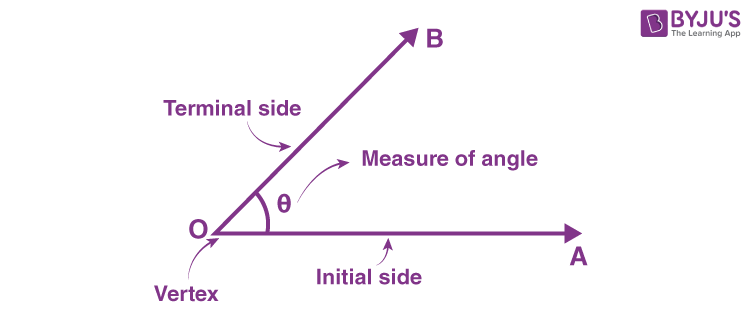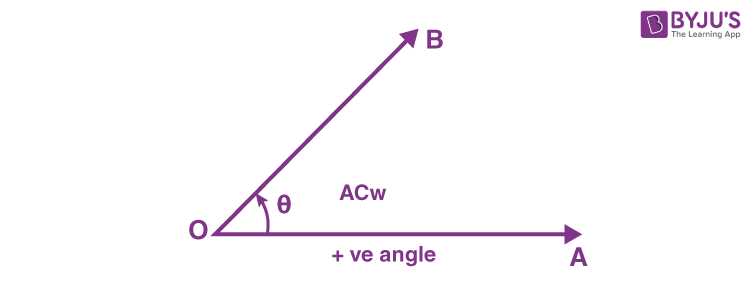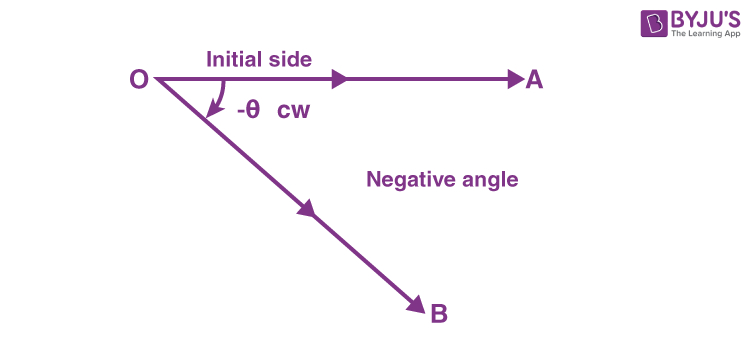Win up to 100% scholarship on Aakash BYJU'S JEE/NEET courses with ABNAT Win up to 100% scholarship on Aakash BYJU'S JEE/NEET courses with ABNAT

# Angle Measurement

Angle measurement is an important topic in geometry. In this article, students will learn how to find angle measurement, systems for measuring angles, positive and negative angles, conversion and solved examples.

## What Is Angle?

When a ray OA starts from its initial position, it rotates about its endpoint o and takes the final position OB, and then an angle is formed.### Positive and Negative Angles

An angle formed by a rotating ray is said to be positive or negative depending on whether it moves an anticlockwise or a clockwise direction, respectively.## Measurement of Angle

There are three systems for measuring angles:

1. Sexagesimal system
2. Centesimal system
3. Circular system

### Sexagesimal System/Degree Measure

This is also called the English system.

In this system,

1st right angle = 90o

1o = 60’

1’ = 60’’

### Centesimal System of Angle Measurement

This is also known as the French system.

$$\begin{array}{l}{1}^{g} = {100}’\\ {1}’ = {100}”\end{array}$$

### Circular System of Angle Measurement

This is very popularly known as the radian system.

In this system, the angle is measured in radians.

• A radian is an angle subtended at the centres of a circle by an arc, and the whole length is equal to the radius of the circle.

Note:

The number of radians in an angle subtended by an arc of the circle at the centres is equal to the arc/radius

$$\begin{array}{l}\theta =\frac{arc}{radius}\end{array}$$

Important Conversions:

1. $$\begin{array}{l}\pi \,radian=180{}^\circ\end{array}$$
2. $$\begin{array}{l}1\,radian=\frac{180}{\pi }\end{array}$$
3. $$\begin{array}{l}1{}^\circ =\left( \frac{\pi }{180} \right)\ rad\end{array}$$
4. If D is the number of degrees, R is the number of radians, and G is the number of grades in angle θ, then
$$\begin{array}{l}\frac{D}{90}=\frac{G}{100}=\frac{2R}{\pi }\end{array}$$
5. θ = 1/r, where θ = angle subtended by an arc of length 1 at the centre of the circle.
6.  Some of the standard radian to degree conversions are given below:

### Related Videos:

Different Types of AnglesDraw Angles### Practice Problems

Illustration 1:

Write (3.25) o in D-M-S

Solution: 3o– 0.25 × 60’ = 3o15’

Illustration 2:

Write in (12.3456)g G- M- S

Solution:

$$\begin{array}{l}{{12}^{g}}-34′-56^{”}\end{array}$$

Illustration 3:

Solution:

$$\begin{array}{l}30{}^\circ \times\frac{\pi }{180}=\frac{\pi }{6}\end{array}$$
 Remember $$\begin{array}{l}{{\pi }^{c}}=180\end{array}$$ $$\begin{array}{l}{{1}^{c\,}}=\frac{180}{\pi }=\frac{180}{22}\times 7\,=\,57{}^\circ 16’22^{”}\end{array}$$ $$\begin{array}{l}{{1}^{c\,}}\simeq 57{}^\circ\end{array}$$

Illustration 4:

If the angles of a triangle are in the ratio 1 : 2 : 3, then find the angles in degrees.

Solution:

Let the angles be x, 2x and 3x.

Then, x + 2x + 3x = 180°    ….[∵ sum of the angles of a triangle = 180°]

∴ 6x = 180°

∴ x = 30°, 2x = 60° and 3x = 90°

Illustration 5:

Which of the following pairs of angles are not co-terminal?
(A) 330°, − 60°

(B) 405°, − 675°

(C) 1230°, − 930°

(D) 450°, − 630°

Solution:

Here, 405° − (− 675°) = 1080° = 3(360°),
1230° − (− 930°) = 2160° = 6(360°)
and 450° − (− 630°) = 1080° = 3(360°) are a multiple of 360°.

Hence, these angles are co-terminal.
Now, 330° − (− 60°) = 390° which is not a multiple of 360°.

So, these pair of angles are not co-terminal.

Illustration 6:

If the arcs of the same length of two circles subtend 75° and 140° at the centre, then the ratio of the radii of the circles is _____.

Solution:

S1 = S2
∴ r1 × θ1 = r2 × θ2
∴ r1 [75π / 180] = r2 [140 π / 180] ∴ r1 / r2 = 140 / 75
∴ r1 / r2 = 28 / 15

Illustration 7:

The perimeter of a sector of a circle, of area 36π sq.cm., is 28 cm. What is the area of the sector?

Solution:

Area of circle = πr2 = 36π sq.cm
∴ r = 6 cm
Now, the perimeter of sector = 2r + S
But, the perimeter is given to be 28 cm.
∴ 28 = 12 + S
∴ S = 16 cm
Thus, the area of sector = (1/2) × r × S = (1/2) × 6 × 16 = 48 sq.cm

Q1

### What do you mean by a radian?

A radian is defined as the ratio of the length of the arc that the angle subtends to a circle, divided by the length of the radius of the same circle.

Q2

### What is the best way of measuring an angle?

The best way to measure an angle is to use a tool named protractor.

Q3